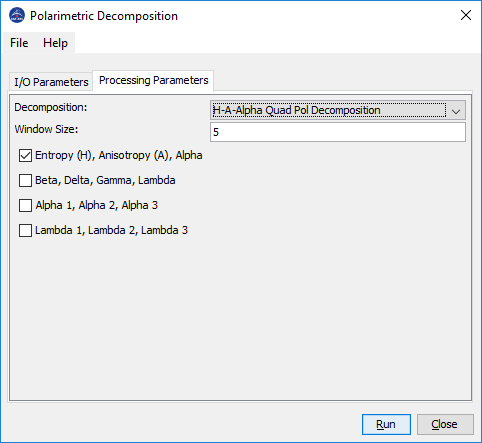# H-Alpha Dual Pol Decomposition - too many output bands?

Hello,
I applied H-Alpha decomposition to dual-pol TerraSAR-X data. As an output I got a .dim product with 3 bands: entropy, anisotropy and alpha. It seems like it’s not correct as the 2x2 coherence matrix can be decomposed into two eigenvalues while for computing the anisotropy the second and the third eigenvalues are used (that means 3x3 matrix is needed).
Thanks in andvance for any comments.

Hi:
I think the parameter A is calculated using two eigenvalues of C2 matrix, the definition of this parameter should be modified when the input is dual-polar data.

I have another question on this.

Does anyone have a literature reference where Alpha1, Alpha2, Alpha3, Lambda1, Lambda2 and Lamdba3 are explained? The the other parameters are quite clear to me but I couldn’t find something in Lee/Pottier (2009) Polarimetric radar imaging. Maybe I am searching at the wrong location or those are named differently.
According to the “About” section, @junlu and @lveci developed this module. I would be grateful for any hint.In H-A-Alpha decomposition, we first perform eigenvalue decomposition of the T3 matrix. The three eigenvalues we obtained are Lambda1, Lambda2 and Lamdba3. Then we compute Alpha1, Alpha2 and Alpha3 from the three corresponding eigenvectors. Finally we compute Alpha by the weighted average of Alpha1, Alpha2 and Alpha3 where the weights are the probabilities computed using Lambda1, Lambda2 and Lamdba3.

2 Likes

I see, thank you very much!

If nobody has a need for those outputs, we could remove them to avoid confusion.

I currently test PolSAR features in a classification of urban dwelling density. If they turn out to have an impact on the result (feature ranking) they would be nice side products. I would expect that Alpha and Lambda have higher importance, but I’ll let you know.

Hello, everybody! I wander know that if the ‘window size’ means the size of the despeckle window??

Yes, the window is for computing the mean coherency matrix for each pixel

Does the “H-Alpha dual-pol decomposition” operation is workable for a dual-pol Sentinel 1 SLC data. Or is this operation only valid for a C2 matrix created from a Compact-pol data (RH/RV or LH/LV) ??